## Tuesday, August 9, 2016

### Rotating the daughter edges of a multifurcation in all possible ways

Related to what I have been working on, the following is a function to compute all possible rotations around a multifurcating node. The function is simple - because all that is required is that we reorder the edges of leading to the daughters descended from that node in all possible ways (something that can be done using `permn` in the combinat package), and then (if we are inclined) “untangle” the tree by reordering the edges or writing & reading it to/from a string.

Note that the number of possible rotations around a multifurcating node (excluding the present rotation) is equal to n!-1 (n-factorial minus one, the original rotation). This is 5 for a trifurcation, 24 for a quadrifurcation, and so on.

``````## load packages
library(combinat)
library(phangorn)
library(phytools)

nodelabels()
``````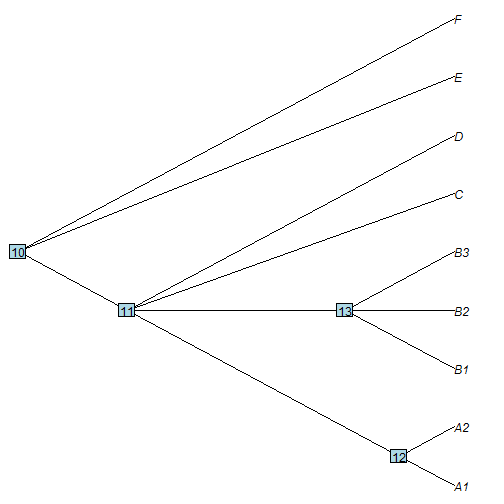``````## our function
rotate.multi<-function(tree,node){
kids<-Children(tree,node)
if(length(kids)>2){
ii<-sapply(kids,function(x,y) which(y==x),y=tree\$edge[,2])
jj<-permn(ii)
foo<-function(j,i,t){
t\$edge[i,]<-t\$edge[j,]
if(!is.null(t\$edge.length))
t\$edge.length[i]<-t\$edge.length[j]
}
obj<-lapply(jj[2:length(jj)],foo,i=ii,t=tree)
class(obj)<-"multiPhylo"
obj
}
``````

Now, let's try it:

``````## around node 13
trees<-rotate.multi(tree,13)
par(mfrow=c(3,2))
par(mar=c(0.5,0,0.5,0))
``````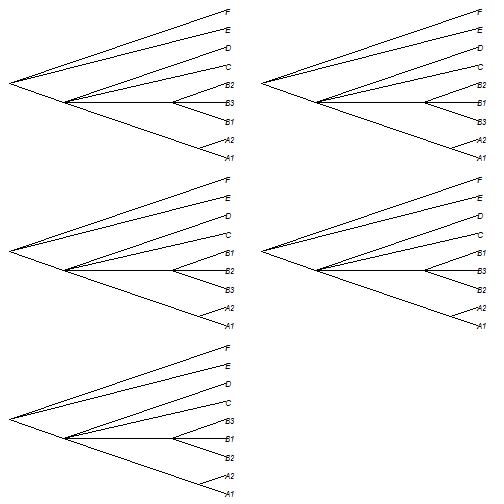``````## around node 11
trees<-rotate.multi(tree,11)
par(mfrow=c(5,5))
par(mar=c(0.5,0,0.5,0))
``````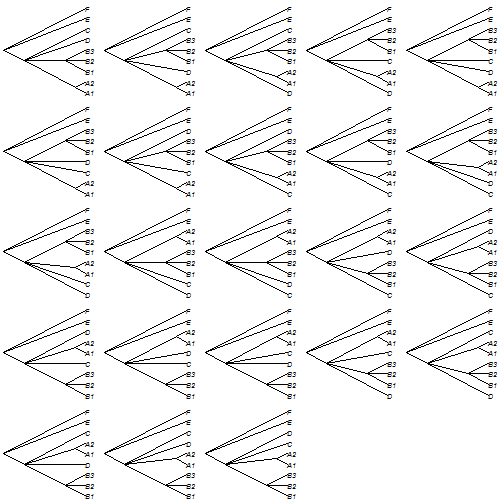This also works when the tree has edge lengths - though if it has any other element or attribute in edge order, there may be some problems!

E.g.:

``````tree\$edge.length<-runif(n=nrow(tree\$edge))
plotTree(tree)
``````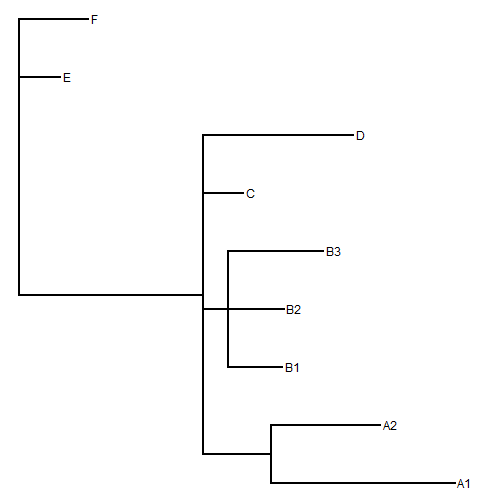``````par(mfrow=c(5,5))
plotTree(rotate.multi(tree,11))
``````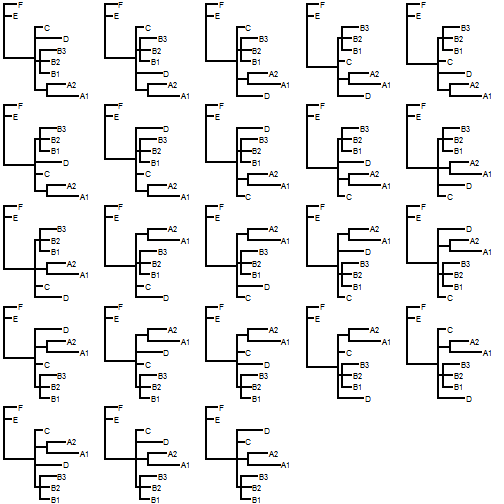That's pretty much it, though I also wanted to record for posterity (and in case I need it later), this vectorized version of `multi2di` for objects of class `"multiPhylo"`.

``````MULTI2DI<-function(x){
obj<-lapply(x,multi2di)
class(obj)<-"multiPhylo"
obj
}
``````